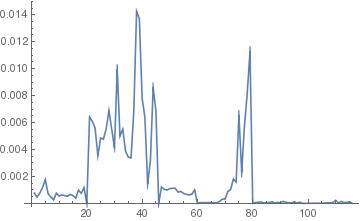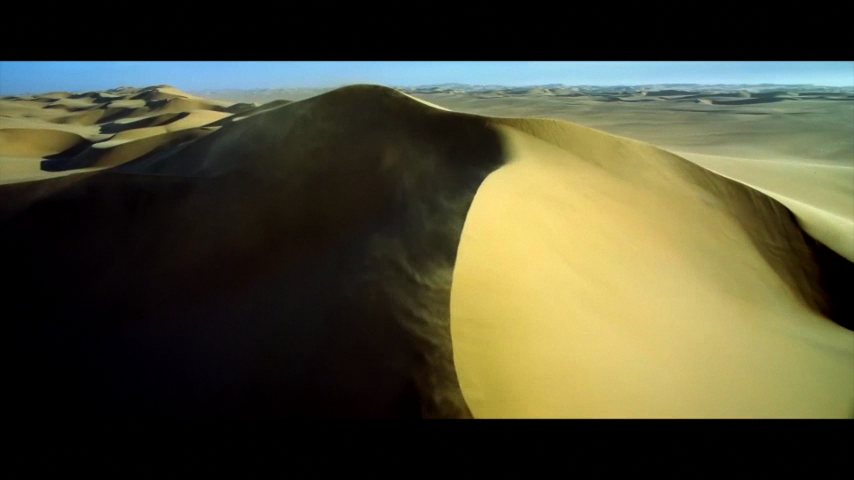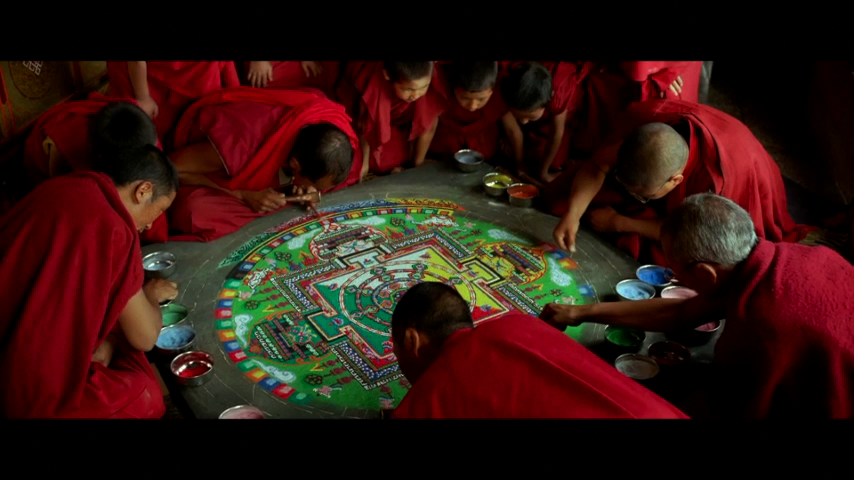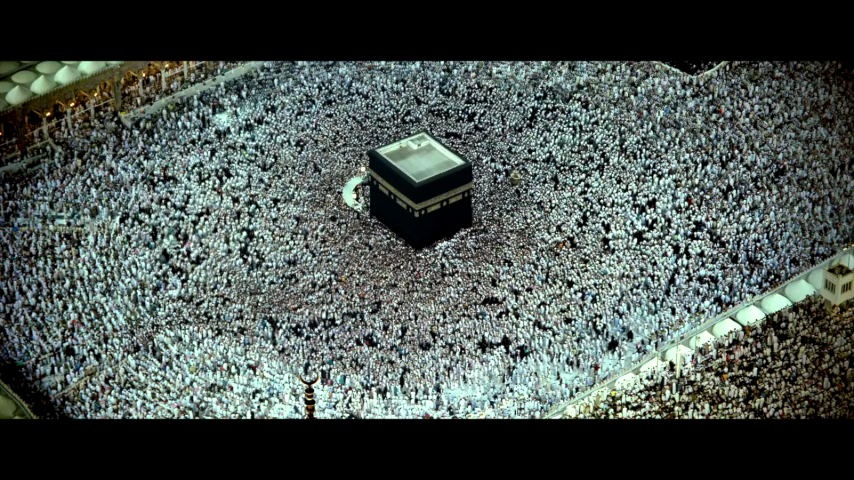# Curvature of Movies

We took here a few seconds from the movie Samsara. In order to keep the computations in a reasonable range, we scaled the individual frames of the picture to 854x480 pixels and took a frame only every 1/8 seconds. Now we have a matrix-valued curve r: [0,T] to the linear space M(480 x 854) of 480 x 854 matrices. [As each pixel has a Red,Green and Blue value, the mathematica program actually processed matrices with 3*854=2562 columns, that is we work in the vector space M(480 x 2562). It is a amazing that the actual movie when seen in full 4K resolution (3840 x 2160 pixels) shows a path r(t) in M(2160 x 11520) which means 24 Million Pixels. If each color is represented as a integer between 0 and 255 (8 bit=1Byte) leading to 24-bit true color, then each color pixel has one Byte and a frame has 24 Million Bytes = 24 Mega Bytes. In our case, with the reduced version (we used video conversion to scale the original high resolution movie down to the small matrices), each picture frame has uncompressed only 1 MegaByte. The pictures were kept in loss-less compressed PNG format of roughly 500 KBytes. If compressed down (with loss) like to JPG, a frame is about 100 K only. It is typical that image compression can bring down the size by about 1/10. This is Fourier theory in action. Now for a movie, where we also have a time component, compression can also be applied time wise and produce dramatic more compression as much of a picture does not change from one frame to an other. For Samsara, which is 102 minutes =6120 seconds long, assuming 30 frames per second, there are 183600 frames meaning 4568555520000 Bytes = 4.5 TBytes. This means that storing Samsara completely uncompressed as matrices would fill out a modern 5 TBytes harddrive. It indeed can happen in movie processing software like Final Cut or Premiere, that one deals with hundreds of Gigabytes of data at the same time, when working on a movie of that length in full 4K resolution. There is still some compression used for the individual frames, the movie software only keeps a local parts of the movie uncompressed.] We can now compute the curvature |T'|/|r'|, where T=r'/|r'| is the unit tangent vector. In our case, with a discrete time, we take r'(t) = r(t+1)-r(t) which is the difference between the picture at time t+1 and time t. The dot product between two matrices has been introduced in the first unit of this course. It is tr(AT B) = sumi,j Aij Bij (a formula you verified in the first homework). We have then a length |A| and can so define the curvature using the usual formula K = |T'|/|r'|. We had 116 frames (as the movie piece is about 14.25 seconds long and 14.25*8=116) and can plot the curvature K(t):Direct Media Links: Webm, Ogg Ipod ;